# Electronics: RC circuits and Exponential Charging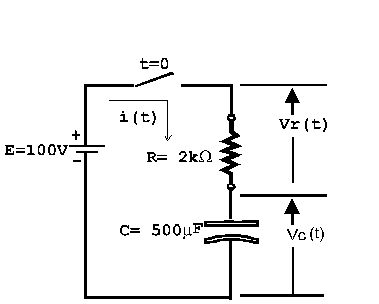An RC circuit is one that contains a resistor and capacitor. Consider the RC circuit shown in the figure. Suppose that the switch is closed at time t = 0 s and that the capacitor has no initial stored charge on it. Once the switch closes, a current will begin flowing in the circuit. This current will deposit charge on the capacitor, leading to a voltage vC(t) across the capacitor.

The current i(t) is given by the formula: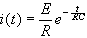(equation 1)

for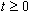where i is the current (in amperes), E is the battery voltage (in volts), R is the resistance (in Ohms, Ω), C is the capacitance (in Farads, F) and t is time (in seconds, s).

## Problem:

1. Find a formula for the capacitor voltage vC(t).
2. Given that E, R and C have the values shown in the diagram, find the capacitor voltage 2 seconds after the switch is closed.
3. How long after the switch is closed will the capacitor voltage be equal to 70% of its final value?

## Solution:

From Kirchhoff&#39;s voltage law we know that: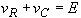(equation 2)

Then from Ohm&#39;s law we have: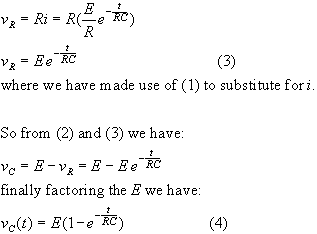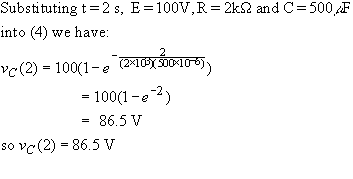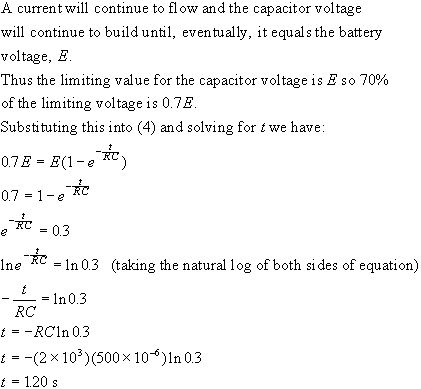Thus the capacitor voltage reaches 70% of maximum after 1.20s.Written by Jim Waterman, October 6, 1997